Home > CC4 > Chapter A > Lesson A.1.8 > ProblemA-83

A-83.

Simplify each of the following equations and solve for the variable. Show all work and check your solution, if possible. Homework Help ✎

1. 3x − 7 + 9 − 2x = x + 2

2. −2m + 8 + m + 1 = 0

3. 2 = x + 6 − 2x

4. 0.5p = p + 5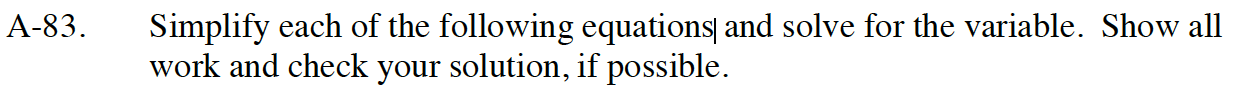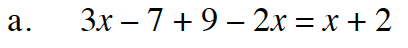Combine like terms.

x + 2 = x + 2

Subtract 2 from both sides.

x + 2 = x + 2
−2 −2

x = x

Any number makes this true.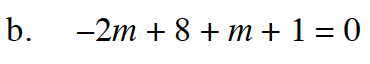Follow the steps in part (a).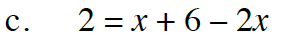Follow the steps in part (a).

x = 4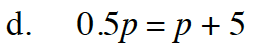Move all the variables to one side.

Follow the steps in part (a).